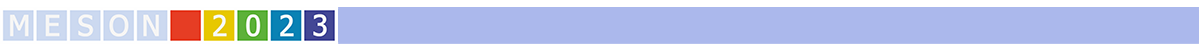#MESON2023

Jun 22 – 27, 2023
Auditorium Maximum
Europe/Warsaw timezone

## $\eta_c$ production within light-cone approach in $pp$ and $e^{-}\, A$ collisions

Jun 23, 2023, 5:30 PM
20m
Medium lecture hall (A) (Auditorium Maximum)

Parallel

### Speaker

Izabela Babiarz (Institute of Nuclear Physics PAS)

### Description

The charmonium state $\eta_{c}$ is the heavy pseudoscalar meson ($J^{PC} = 0^{-+}$) lied under $D\bar D$ threshold. We will review the proton-proton and $e^- A$ reactions, where $\eta_{c}$ can be produced through the two virtual gluons or one virtual photon.
The main ingredient in our light-cone approach is the space-like transition form factor with dependence on two virtualities of the fused particles.
The idea is to construct the form factor on the basis of the wave function of the $c\bar c$ state. The radial part of the wave function can be found by solving the Schrödinger equation then "translation" to the light-cone variable is performed using the Terentev prescription.
I will present the effects of the so-obtained form factors in the context of proton-proton collisions by taking into account the proper color factors and coupling constant for the off-shell gluon-gluon transition to the pseudoscalar meson. We have compared our results to LHCb data for the prompt production of $\eta_{c}$ at 7 TeV, 8 TeV and 13 TeV c.m. energies.
Future facilities such as EIC or LHeC give the opportunity to probe the form factor dependence on the virtuality in $e^- A$ collisions. I will present results for the photon $Q^2$-dependence within the equivalent photon approximation.

Based on the references
I.~Babiarz, V.~P.~Goncalves, R.~Pasechnik, W.~Schäfer and A.~Szczurek,
"${\gamma^* \gamma^* \to \eta_c (1S,2S)}$ transition form factors for spacelike photons",\
Phys. Rev. D \textbf{100} (2019) no.5, 054018, doi:10.1103/PhysRevD.100.054018, [arXiv:1908.07802 [hep-ph]].\
I.~Babiarz, R.~Pasechnik, W.~Schäfer and A.~Szczurek,
"Prompt hadroproduction of $\eta_c(1S,2S)$ in the $k_T$-factorization approach",\
JHEP \textbf{02} (2020), 037, doi:10.1007/JHEP02(2020)037,[arXiv:1911.03403 [hep-ph]].\
I.~Babiarz, V.~P.~Goncalves, W.~Schäfer and A.~Szczurek,\
"Exclusive $\eta_c$ production in electron-ion collisions", work in progress

### Primary author

Izabela Babiarz (Institute of Nuclear Physics PAS)

### Co-authors

Antoni Szczurek (Institute of Nuclear Physics PAS) Wolfgang Schafer (Institute of Nuclear Physics PAS)